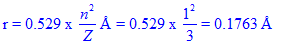# RADIUS OF BOHR'S nth ORBIT  - FORMULA & RATIOS - SOLVED PROBLEMS IIT JEE - NEET - CSIR UGC NET - GATE CHEMISTRY

First of all, watch the following video on radius of Bohr's orbit.

## Which hydrogen like species will have same radius as that of Bohr's first orbit of hydrogen atom?

(IIT JEE 2004)

1) n=2, Li2+

2) n=2, Be3+

3) n=2, He+

4) n=3, Li2+

Logic:

Radius of Bohr's orbit in hydrogen and hydrogen like species can be calculated by using the following formula.Where

n = prinicipal quantum number of orbit.

Z = atomic number

Now compare the n2/Z values of orbits for given species with that of hydrogen's first orbit to get the answer.

Solution:

 1st orbit of H atom Option 1 n=2, Li2+ Option 2 n=2, Be3+ Option 3 n=2, He+ Option 4 n=3, Li2+ n = 1 2 2 2 3 Z = 1 3 4 2 3 n2/Z 12/1 = 1 22/3 = 4/3 22/4 = 1 22/2 = 2 32/3 = 3

Conclusion:

Since the n2/Z values are same for second orbit of Be3+ and first orbit of H, their radii are also equal. Answer is option 2.

## Related questions

### 1) The radius of Bohr’s first orbit in Li2+ is:

1) 0.0587 pm

2) 17.63 pm

3) 176.3 pm

4) 0.529 pm

Logic:

Use the following equation to calculate the radius in Angstroms, Å.Then convert the value into picometers.

Remember 1 Å=102 pm

Solution:In picometers, the radius = 0.1763 x 102  = 17.63 pm

### 2) The ratio of radii of first three Bohr's orbits in hydrogen atom is:

1) 1 : 4 : 9

2) 1 : 2 : 3

3) 1 : 2 : 4

4) 9 : 4 : 1

Logic and Solution:

Atomic number, Z is equal to 1.

Hence the radius of nth orbit, rn = 0.529n2 Å.

For first three orbits, n values are 1,2 and 3.

Therefore:

The ratio of radii of first three orbits = r1 : r2 : r3 = n12 : n22 : n32 = 12 : 22 : 32 = 1 : 4 : 9

## Homework

1) What is the ratio of the radii of first orbits of H, He+ and Li2+ ?

2) Is it possible to calculate the radii of orbits in He2+ using Bohr's equation?

3) What is the ratio of circumferences of first three orbits in hydrogen atom?

4) Calculate the radius of 2nd orbit in He+.

5) The radius of which of the following orbit is same as that of first orbit of hydrogen atom....

A) second orbit of He+

B) first orbit of Li2+

C) second orbit of Be3+

D) None

Answer: option C is correct

6) The ratio of radii of first orbits of He+ and Li2+ is...... (3:2)

7) The ratio of radii of first shell of H atom and that of fourth shell of He+ ion is........ (1:8)

8) The ratio of radius of fourth orbit of He+ ion to the radius of second orbit of Be3+ ion is....... (8 : 1)

9) Bohr atomic radius of 3rd shell of Li2+ ion is........( 3 x 0.529 = 1.587 Ao)

10) The atomic radii of first orbit of h-atom is 0.529 Ao. The radii of second orbit of He+ is.........

11) Derive the relationship between the nth bohr radius of hydrogen atom &  Li2+ ion.

Jump to Radial and angular nodes of orbitals  - IIT JEE - NEET - CSIR NET - GATE - IIT JAM solved problems